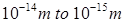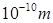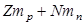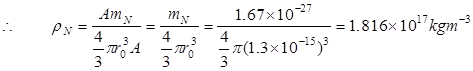# Describe the general properties of nucleus.## General Properties of Nucleus:

### (i) Nuclear Size:

From Rutherford’s work, the mean radius of nucleus isand the radius of the atom is. Thus the radius of the nucleus is 10,000 times smaller than the radius of the atom.

The empirical formula for the nuclear radius is,

Here, A is the mass number,is a constant.

From this formula the radius of the Carbon () nucleus is.

### (ii) Nuclear Mass:

If there are Z protons and N neutrons then the assumed mass is,

Where,is the mass of each proton andis mass of each neutron. But,

Real nuclear mass <Difference of the masses,

Δm is called the mass defect.

### (iii) Nuclear Density:

Here, nuclear mass =, where A is the mass number andis the mass of the nucleon =### (iv) Nuclear Charge:

The charge of the nucleus is the charge of the proton contained in it. Each proton has a positive charge ofThe nuclear charge is Ze, where Z is the atomic number of the nucleus.